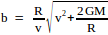## Guest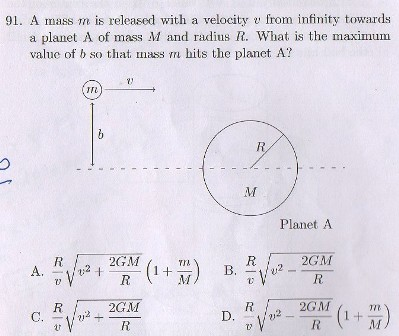7 years ago
p { margin-bottom: 0.25cm; line-height: 120%; }

We can use law of conservation of angular momentum and law of conservation of mechanical energy to solve this problem.

Initial angular momentum Li = mvb

Final angular momentum Lf = mVR (assuming that the mass m will just graze the surface of earth, as per requirement of the problem statement).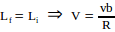Initial Potential Energy Ui = 0

Final Potential Energy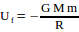Initial Kinetic Energy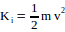Final Kinetic Energy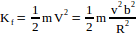Substituting the values in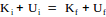and solving for b,

we get